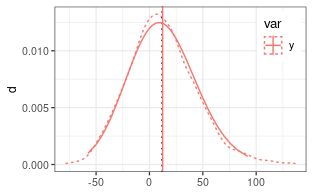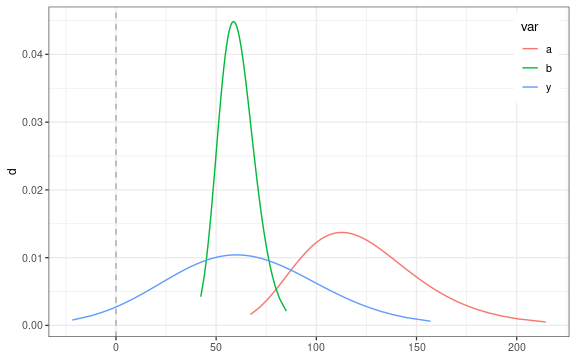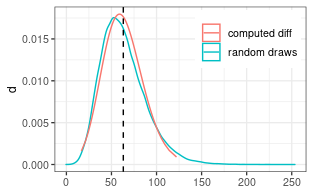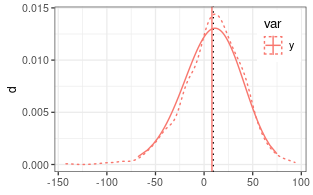# Approximating the difference of lognormal random variables

Lo 2012 reports an approximation for the difference of two random variables by a shifted lognormal distribution.

Rather than approximating the density of $$y = a - b$$, it approximates the density of $$y_s = a - b + s$$, where $$s$$ is the shift. Hence, one has to subtract $$s$$ from provided mean and quantiles. One can can use the variance, and relative error but has to recompute the relative error.

## Two uncorrelated random variables

# generate nSample values of two lognormal random variables
mu1 = log(110)
mu2 = log(100)
sigma1 = 0.25
sigma2 = 0.15
#(coefDiff <- estimateSumLognormal( c(mu1,mu2), c(sigma1,sigma2) ))
(coefDiff <- estimateDiffLognormal(mu1,mu2, sigma1,sigma2, 0))
##           mu        sigma        shift
##   6.14704349   0.06859943 456.07414434
(expDiff <- getLognormMoments(coefDiff["mu"], coefDiff["sigma"])[,"mean"] -
coefDiff["shift"])
##     mean
## 12.36042

Several functions accept the shift argument to handle this already.

getLognormMoments(coefDiff["mu"], coefDiff["sigma"], shift = coefDiff["shift"])
##          mean     var       cv
## [1,] 12.36042 1035.05 2.602839
getLognormMode(coefDiff["mu"], coefDiff["sigma"], shift = coefDiff["shift"])
##       mu
## 9.065469
getLognormMedian(coefDiff["mu"], coefDiff["sigma"], shift = coefDiff["shift"])
##       mu
## 11.25952

For the functions from the stats package, the shifting has to be done manually.

p <- seq(0,1,length.out = 100)[-c(1,100)]
dsPredY <- data.frame(
var = "y",
q_shifted = qlnorm(p, coefDiff["mu"], coefDiff["sigma"] )
) %>%
mutate(
q = q_shifted - coefDiff["shift"],
d = dlnorm(q_shifted, coefDiff["mu"], coefDiff["sigma"])
)

A check by random numbers (dotted lines) shows close correspondence.## Test if difference is significantly different from zero

The probability of the zero quantile needs to be larger than a significance level. We can compute it based on the lognormal approximation.

Since Lo12 is only accurate if the expected difference is small compared to the expected sum, the probability of the difference being larger than zero can be estimated by a sampling both terms.

  mu1 = log(120)
mu2 = log(60)
sigma1 = 0.25
sigma2 = 0.15
coefDiff <- estimateDiffLognormal( mu1,mu2,sigma1,sigma2, corr = -0.8 )
## Warning in estimateDiffLognormal(mu1, mu2, sigma1, sigma2, corr = -0.8):
## Expected S0+/S0- << 1 but this ratio was 0.34219239671082. The Lo 2012
## approximation becomes inaccurate for small numbers a and b.
  pLo <- plnorm(0 + coefDiff["shift"], coefDiff["mu"], coefDiff["sigma"])
pSample <- pDiffLognormalSample(mu1,mu2,sigma1,sigma2, corr = -0.8)
## Loading required namespace: mvtnorm
  c(pLo = as.numeric(pLo), pSample = pSample)
##        pLo    pSample
## 0.04540137 0.03449000

In the example both approaches give a probability of less than 5% so that we conclude that the difference is significant.## Two positively correlated variables

if (!requireNamespace("mvtnorm")) {
warning("Remainder of the vignette required mvtnorm installed.")
knitr::opts_chunk\$set(error = TRUE)
}
corr = 0.8
(coefDiff <- estimateDiffLognormal(mu1,mu2, sigma1,sigma2, corr = corr))
## Warning in estimateDiffLognormal(mu1, mu2, sigma1, sigma2, corr = corr):
## Expected S0+/S0- << 1 but this ratio was 0.34219239671082. The Lo 2012
## approximation becomes inaccurate for small numbers a and b.
##          mu       sigma       shift
##   5.1762267   0.1264911 115.3050125
(expDiff <- getLognormMoments(
coefDiff["mu"], coefDiff["sigma"], shift = coefDiff["shift"])[,"mean"])
##    mean
## 63.1304

Check with sampled distribution.

nSample <- 1e5
sigma_vec = c(sigma1, sigma2)
corrM <- setMatrixOffDiagonals(
diag(nrow = 2), value = corr, isSymmetric = TRUE)
covM <- diag(sigma_vec) %*% corrM %*% diag(sigma_vec)
xObsN <- exp(mvtnorm::rmvnorm(nSample, mean =  c(mu1, mu2), sigma = covM))
head(xObsN)
##           [,1]     [,2]
## [1,]  98.52797 54.44233
## [2,] 139.01873 61.70344
## [3,] 116.34407 56.07350
## [4,] 161.93879 75.96263
## [5,] 154.22407 68.35834
## [6,]  98.16661 51.82886
y = xObsN[,1] - xObsN[,2]The approximation for the difference of positively correlated random numbers predicts a narrower distribution than with the uncorrelated or negatively correlated difference above. However, this case less accurate and shows some deviations from the sampled distribution around the mode.

## Subtracting a variable with larger variance

The method of Lo12 requires $$\sigma_b < \sigma_a$$ and otherwise gives an error.

# generate nSample values of two lognormal random variables
mu1 = log(110)
mu2 = log(100)
sigma1 = 0.15
sigma2 = 0.25
try(coefDiff <- estimateDiffLognormal(mu1,mu2, sigma1,sigma2, 0))
## Error in estimateDiffLognormal(mu1, mu2, sigma1, sigma2, 0) :
##   expected sigma_a > sigma_b but got 0.15 <= 0.25. Exchange the terms and negate the resulting quantiles (see vignette('lognormalDiff').

But one can compute the density of $$y_r = -y = b - a$$ and plot the density of the shifted and negated distribution.

# note the switch of positions of mu1 and mu2: mu2 - mu1
#(coefDiff <- estimateDiffLognormal(mu1,mu2, sigma1,sigma2, 0)
(coefDiff <- estimateDiffLognormal(mu2,mu1, sigma2,sigma1, 0))
##           mu        sigma        shift
##   6.10147970   0.06859943 455.64000921
# note the minus sign in front
(expDiff <- -(getLognormMoments(
coefDiff["mu"], coefDiff["sigma"], shift = coefDiff["shift"])[,"mean"]))
##     mean
## 8.070146
p <- seq(0,1,length.out = 100)[-c(1,100)]
dsPredY <- data.frame(
var = "y",
q_shifted_neg = qlnorm(p, coefDiff["mu"], coefDiff["sigma"] )
) %>%
mutate(
)Because we subtract a large-variance lognormal variable, the distribution becomes right-skewed.